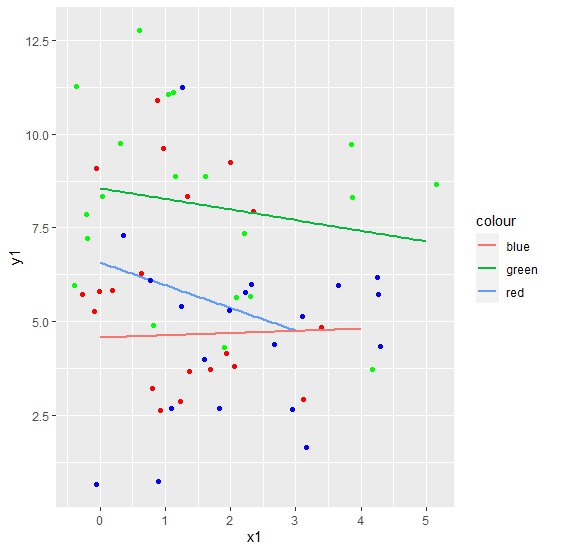# Create multiple regression lines in a single plot using ggplot2 in R.

To create multiple regression lines in a single plot using ggplot2, we can use geom_jitter function along with geom_smooth function. The geom_smooth function will help us to different regression line with different colors and geom_jitter will differentiate the points.

Check out the below Example to understand how it can be done.

## Example

Following snippet creates a sample data frame −

x1<-rpois(20,1)
y1<-rpois(20,5)
x2<-rpois(20,2)
y2<-rpois(20,8)
x3<-rpois(20,2)
y3<-rpois(20,4)
df<-data.frame(x1,y1,x2,y2,x3,y3)
df

The following dataframe is created

  x1 y1 x2 y2 x3 y3
1 2  2  0  6  1 6
2 3  4  0  9  1 7
3 2  4  3  7  2 3
4 0 12  2 11  0 1
5 0  2  0  6  1 1
6 1  7  2  7  1 3
7 0  4  0  4  1 5
8 0  3  2  5  0 1
9 1  4  3  3  0 9
10 0  2  0  8  3 5
11 0  7  4 11  2 4
12 0  4  3  8  2 1
13 0  6  0  6  2 4
14 1  6  1  9  2 2
15 2  3  1  9  6 2
16 1  3  1 10  5 2
17 0  5  1  8  2 6
18 1  2  4  7  2 4
19 0  5  2 11  0 7
20 2  8  4  8  2 4

To load the ggplot2 package and create regression lines for multiple models in single plot on the above created data frame, add the following code to the above snippet −

x1<-rpois(20,1)
y1<-rpois(20,5)
x2<-rpois(20,2)
y2<-rpois(20,8)
x3<-rpois(20,2)
y3<-rpois(20,4)
df<-data.frame(x1,y1,x2,y2,x3,y3)
library(ggplot2)

ggplot(df)+geom_jitter(aes(x1,y1),colour="red")+geom_smooth(aes(x1,y1,col="red"
),method="lm",se=FALSE)+
+
geom_jitter(aes(x2,y2),colour="green")+geom_smooth(aes(x2,y2,col="green"),metho
d="lm",se=FALSE)+
+
geom_jitter(aes(x3,y3),colour="blue")+geom_smooth(aes(x3,y3,col="blue"),method=
"lm",se=FALSE)
geom_smooth() using formula 'y ~ x'
geom_smooth() using formula 'y ~ x'
geom_smooth() using formula 'y ~ x'

## Output

If you execute all the above given snippets as a single program, it generates the following Output −